# GMAT Math : DSQ: Calculating the surface area of a prism

## Example Questions

### Example Question #1 : Prisms

What is the volume of a cardboard box with six rectangular surfaces?

Statement 1: The length and width are each half the height.

Statement 2: The height is ten inches longer than the width.

BOTH statements TOGETHER are sufficient to answer the question, but NEITHER statement ALONE is sufficient to answer the question.

EITHER statement ALONE is sufficient to answer the question.

Statement 1 ALONE is sufficient to answer the question, but Statement 2 ALONE is NOT sufficient to answer the question.

BOTH statements TOGETHER are insufficient to answer the question.

Statement 2 ALONE is sufficient to answer the question, but Statement 1 ALONE is NOT sufficient to answer the question.

BOTH statements TOGETHER are sufficient to answer the question, but NEITHER statement ALONE is sufficient to answer the question.

Explanation:

To know the volume of the box, which is shaped like a rectangular prism, you multiply the length, the width, and the height together. Neither statement alone gives you those dimensions, just clues as to how they are related. But together, you can form a system of linear equations using height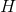and width (and length)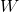.

By the first and second statements, respectively,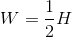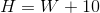This system can be solved: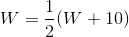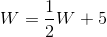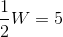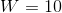From this, you can determine the length and height, and, subsequently, the volume.

### Example Question #2 : Prisms

A box with equal length, width, and height has the following dimensions.

I) The diagonal of the cube is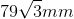.

II) The volume of the cube is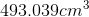.

What is the surface area of the box?

Statement II is sufficient to answer the question, but statement I is not sufficient to answer the question.

Either statement is sufficient to answer the question.

Both statements are needed to answer the question.

Statement I is sufficient to answer the question, but statement II is not sufficient to answer the question.

Either statement is sufficient to answer the question.

Explanation:

To find surface area, we need to know the side length of the box.

We are told the box has equal length, width and height. This means it is a cube.

I) Gives us the diagonal of the cube, from this we can find the side length.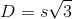, where s is the side length.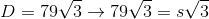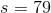Now we can use the side length to find the surface area of the box.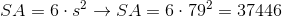II) Gives us the volume of the cube, from which we can also find the side length.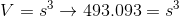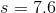From here we can find the surface area.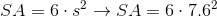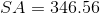Thus, either statement is sufficient.

### Example Question #3 : Prisms

Fry recently met a robot known for bending things. The robot wants to make a box out of steel by bending a single sheet of metal. Find the total area of the sheet of metal given the following:

I) The box will be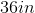long.

II) The box's height will be 2 feet taller than its length, and the box's width will be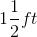less than its length.

Statement I is sufficient to answer the question, but statement II is not sufficient to answer the question.

Statement II is sufficient to answer the question, but statement I is not sufficient to answer the question.

Either statement is sufficient to answer the question.

Both statements are needed to answer the question.

Both statements are needed to answer the question.

Explanation:

Fry recently met a robot known for bending things. The robot wants to make a box out of steel by bending a single sheet of metal. Find the total area of the sheet of metal given the following:

I) The box will belong

II) The box's height will be 2 feet taller than its length, and the box's width will beless than its length.

This question is a surface area question in disguise. To find the surface area of a prism, we need the area of each side. Begin by using I and II to find each side length:

Length: 36 inches or 3 feet

Height: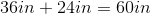or 5 feet

Width: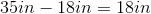or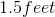So, we have all our side lengths. Next, use the following formula for surface area of a rectangular prism: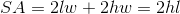Where l,w, and h are length, width and height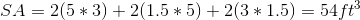Tired of practice problems?

Try live online GMAT prep today.